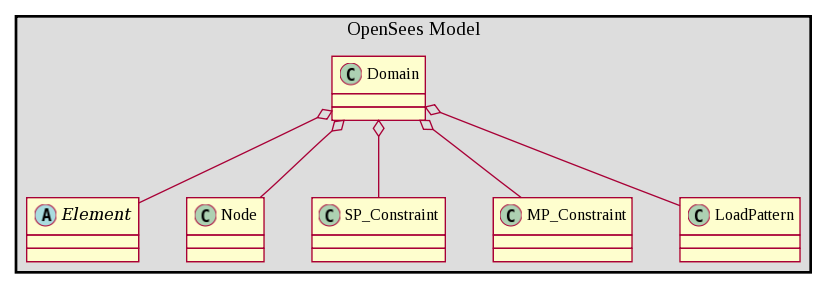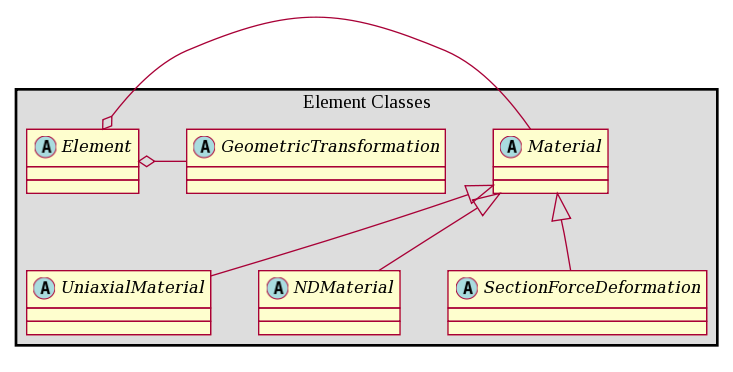# Modeling Commands

The model or domain in OpenSees is a collection (an aggregation in object-oriented terms) of elements, nodes, single- and multi-point constraints and load patterns. It is the aggregation of these components which define the type of model that is being analyzed. The component classes, are as shown in the figure below:The following are the modeling commands that have been added to OpenSees interpreter to create these components of the finite element model:

• model
• element
• node
• SP_Constraint (single-point constraint), which prescribe the movement (typically 0) of a single dof at a node. There are a number of commands for creating single-point coonstraints:
• MP_Constraint (multi-point constraint), which prescribe that the movement of certain dof at one node are defined by the movement of certain dof at another node. There again are a number of commands for defining multi-point constraints.
• timeSeries
• pattern

The following Misc commands are used to generate elements or modify properties of elements or nodes already in the model:

The typical element in OpenSees has the material nonlinearity and sometimes geometric nonlinearities contained in other objects, materials and geometric transformations. Commands have been added to the interpreter to create these objects as well: